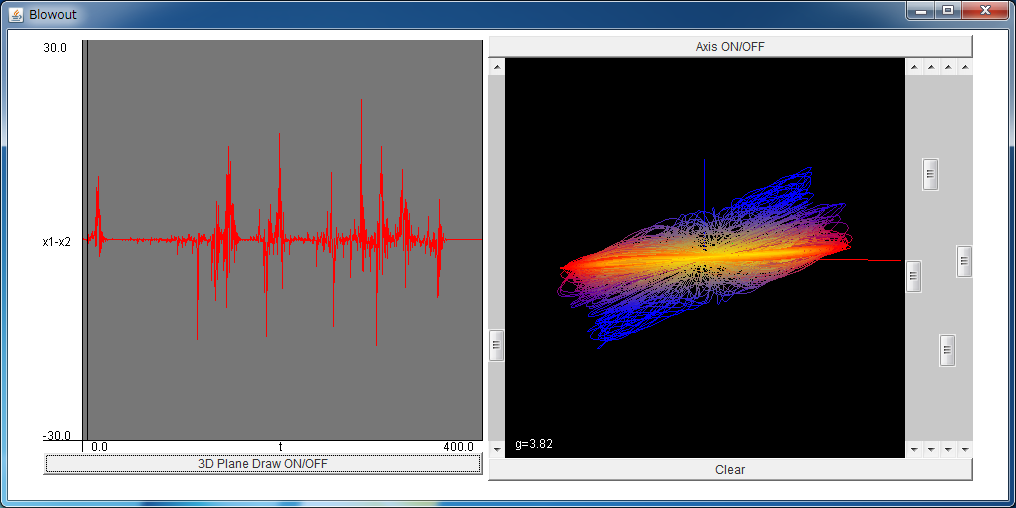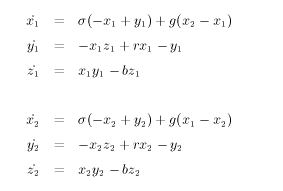# Blowout Bifurcation and On-off IntermittencyIf the above application does not start, please install OpenJDK from adoptium.net.

Let's consider the two coupled Lorenz systems (x1, y1, z1) and (x2, y2, z2) written aswhere g is the coupling strength. The parameters are fixed as sigma=10, r=8/3, and b=28, which are identical values with this page.

When the coupling strength g is sufficiently large, two Lorenz systems synchronize each other, and they form the Lorenz attractor.
Note that the conditions x1=x2, y1=y2, and z1=z2 are satisfied in the synchronized state.

The dimension of the original system is 6, and that of the subspace which satisfies x1=x2, y1=y2, and z1=z2 is 3,
thus the synchronized state can be regard as the state where the system is confined in the 3-dimensional invariant subspace.

The two Lorenz systems desynchronize when the value of g is small,
and this phenomenon is regarded as the destabilization of the confinement within the invariant subspace.
When the confinement within the invariant subspace is destabilized, the blowout bifurcation takes place,
and the intermittent chaos called on-off intermittency is often observed.

In this page, the blowout bifurcation and the on-off intermittency can be observed.
In the righthand of the simulator, the trajectory of the three variables x1, y1, and x1-x2 are shown in the 3-dimensional space,
and each axis is drawn in red, green, and blue, respectively.
The view angle of the 3-dimensional space can be changed with the right four bars.

Note that the (x1, y1) plane is the invariant subspace which confines the system.
When the trajectory of the system is close to the (x1, y1) plane, namely, when the system is close to the invariant subspace,
the trajectory is colored with yellow or red.
On the other hand, when the system is away from the invariant subspace, the trajectory is colored with blue.
With the default parameter, it is observed that the system is sometimes close to the invariant subspace, and sometimes away from it.

The dependence of x1-x2 on time t is shown in the lefthand of the simulator.
The value of x1-x2 sometimes takes the values close to 0 (ON-state), and sometimes takes the non-zero values (OFF-state) intermittently.
Such a property of chaos is called on-off intermittency.
The numerous researchers investigate the properties of on-off intermittency, and it is known that the time series of on-off intermittency is fractal, and its statistics show anomalous behaviors.

When the simulator is started, the coupling strength g is set to the value where the on-off intermittency is observed and the two Lorenz systems transit between the synchronized state and the desynchronized state intermittently.
Let's change the value of g with the bar located in the center of the simulator.
When g is sufficiently large, two Lorenz systems synchronize.
In this case, in the right 3-dimensional space, the trajectory is confined in the (x1, y1) plane, and in the left plane, x1-x2 takes always 0.
When g is changed smaller, the confinement is destabilized at about g=3.9, and this bifurcation is the blowout bifurcation.

The blowout bifurcation takes place when the Lyapunov exponent in the transversal direction of the invariant subspace changes from negative to positive.
Ott and Sommerer discriminated the nonhysteretic blowout bifurcation accompanied by the on-off intermittency with the hysteretic blowout bifurcation accompanied by the riddled basin
The on-off intermittency takes place when the other attractor does not exist outside the invariant subspace, and the riddled basin takes place when the other attractor exists outside the invariant subspace.

Moreover, they conjectured that there will always be either the on-off intermittency or the riddled basin near the typical blowout bifurcation.
It is because the transversal coarse-grained expansion rate is fluctuating.